# Quotient and Remainder Problem type 2 Online Quiz

#### Complete Python Prime Pack

9 Courses     2 eBooks

#### Artificial Intelligence & Machine Learning Prime Pack

6 Courses     1 eBooks

#### Java Prime Pack

9 Courses     2 eBooks

Following quiz provides Multiple Choice Questions (MCQs) related to Quotient and Remainder: Problem type 2. You will have to read all the given answers and click over the correct answer. If you are not sure about the answer then you can check the answer using Show Answer button. You can use Next Quiz button to check new set of questions in the quiz.Q 1 - Divide 243 ÷ 8. Give the quotient and remainder

A - 29 R 3

B - 30 R 1

C - 30 R 3

D - 31 R 3

### Explanation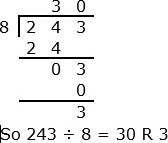Q 2 - Divide 462 ÷ 9. Give the quotient and remainder

A - 50 R 2

B - 50 R 3

C - 51 R 2

D - 51 R 3

### Explanation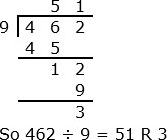Q 3 - Divide 371 ÷ 7. Give the quotient and remainder

A - 53 R 0

B - 53 R 1

C - 53 R 2

D - 53 R 3

### Explanation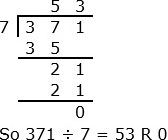Q 4 - Divide 492 ÷ 8. Give the quotient and remainder

A - 60 R 2

B - 60 R 4

C - 61 R 1

D - 61 R 4

### Explanation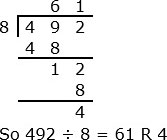Q 5 - Divide 645 ÷ 7. Give the quotient and remainder

A - 92 R 0

B - 92 R 1

C - 92 R 2

D - 92 R 3

### Explanation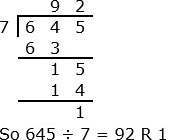Q 6 - Divide 499 ÷ 6. Give the quotient and remainder

A - 82 R 1

B - 82 R 2

C - 83 R 1

D - 83 R 4

### Explanation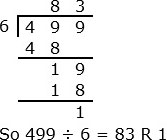Q 7 - Divide 437 ÷ 5. Give the quotient and remainder

A - 87 R 2

B - 87 R 4

C - 88 R 2

D - 89 R 2

### ExplanationQ 8 - Divide 513 ÷ 8. Give the quotient and remainder

A - 63 R 0

B - 63 R 1

C - 64 R 0

D - 64 R 1

### Explanation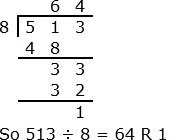Q 9 - Divide 603 ÷ 9. Give the quotient and remainder

A - 66 R 0

B - 66 R 3

C - 67 R 0

D - 67 R 2

### ExplanationQ 10 - Divide 397 ÷ 6. Give the quotient and remainder

A - 66 R 0

B - 66 R 1

C - 67 R 0

D - 67 R 1

### Explanation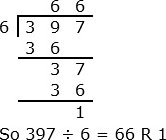quotient_and_remainder_problem_type2.htm